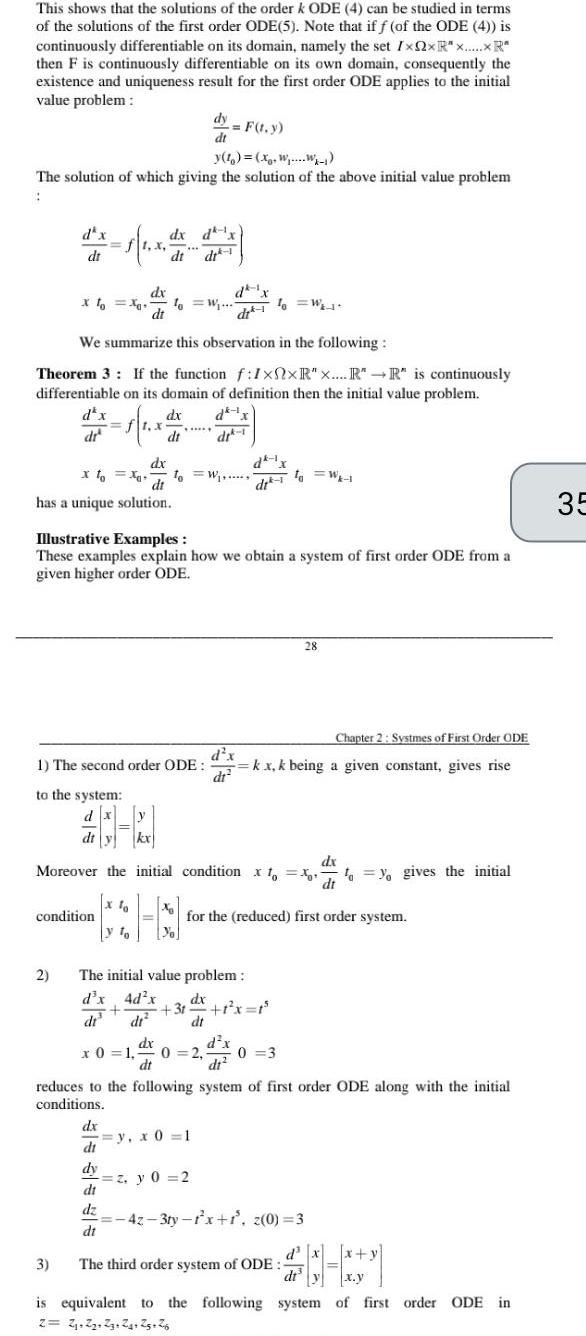Question:

# This shows that the solutions of the order k ODE 4 can be

Last updated: 9/18/2023This shows that the solutions of the order k ODE 4 can be studied in terms of the solutions of the first order ODE 5 Note that if f of the ODE 4 is continuously differentiable on its domain namely the set IxQxR R then F is continuously differentiable on its own domain consequently the existence and uniqueness result for the first order ODE applies to the initial value problem The solution of which giving the solution of the above initial value problem dt to the system dx dt y X dx di has a unique solution 2 dx dt XoXo fo W di We summarize this observation in the following Theorem 3 If the function f 1xxR x R R is continuously differentiable on its domain of definition then the initial value problem dx dk 1x dx 1 x dr dr 1 condition 3 dx dx dt dt dt y To dy F t y dt 1 The second order ODE dx di dy di dz di Illustrative Examples These examples explain how we obtain a system of first order ODE from a given higher order ODE 10 W d x dr kx Moreover the initial condition xoxo yo gives the initial dx The initial value problem d x 4d x dx dr 10 W dr 1 y x 0 1 31 1 x 1 dt d x dt2 z y 0 2 for the reduced first order system dx x 0 1 0 2 0 3 dt to W 1 Chapter 2 Systmes of First Order ODE kx k being a given constant gives rise reduces to the following system of first order ODE along with the initial conditions 28 42 3ty 1 x 1 z 0 3 The third order system of ODE d x x y dt y x y is equivalent to the following system of first order ODE in 2 2 2 23 24 25 26 35